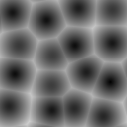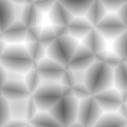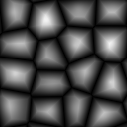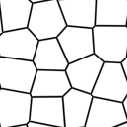SeExpr
User Documentation

#### Variables

The specific variables that are used depends on the context that the expression language is being used in. A common convention we use is to use the following variables in different contexts. These are just examples. Usually the developer of the software using expressions will register the acceptable variables with autocomplete help so that when you type \$ in the expression editor a list of acceptable variables popup.

Image Variables
• \$u, \$v -texture coords (scalars)
• \$Cs, Source image color (vector)
• \$As, Source image alpha (scalar)
• \$Cs, Source image color (vector)
• \$u, \$v -texture coords (scalars)
• \$P - surface point (vector). Note: \$P is sampled from the Pref geometry (if available)
• \$N - surface normal
• \$objectId - per-surface unique object Id, typically a small integer
• \$frame - current frame number
Local Variables

Local variables can be defined at the start of the expression:

\$a = noise(\$P);
\$b = noise(\$a * 1);
pow(\$a, 0.5) + \$b

External variables can also be overridden by local assignment.  This can be useful to scale the noise frequency for instance:

\$P = \$P * 10; # increase noise frequency
fbm(vnoise(\$P) + \$P/4)

float clamp ( float x, float lo, float hi )  - constrain x to range [lo, hi]

float compress ( float x, float lo, float hi )
Compress the dynamic range from [0..1] to [lo..hi]
float expand ( float x, float lo, float hi )
Expand the dynamic range from [lo..hi] to [0..1]
float contrast ( float x, float c )
Adjust the contrast.  For c from 0 to 0.5, the contrast is decreased.  For c > 0.5, the contrast is increased.
float invert ( float x ) - Invert the value.  Defined as 1-x.

float remap ( float x, float source, float range, float falloff, int interp )
General remapping function.  When x is within +/- range of source, the result is one.  The result falls to zero beyond that range over falloff distance.  The falloff shape is controlled by interp. Numeric values or named constants may be used:
int linear = 0
int smooth = 1
int gaussian = 2

color hsi ( color x, float h, float s, float i, float map=1 )
The hsi function shifts the hue by h (in degrees) and scales the saturation and intensity by s and i respectively.  An map may be supplied which will control the shift - the full shift will happen when the map is one and no shift will happen when the map is zero.  The shift will be scaled back for values between zero and one.
color midhsi ( color x, float h, float s, float i, float map, float falloff=1, int interp=0 )
The midhsi function is just like the hsi function except that the control map is centered around the mid point (value of 0.5) and can scale the shift in both directions.  At the mid point, no shift happens.  At 1.0, the full shift happens, and at 0.0, the full inverse shift happens.  Additional falloff and interp controls are provided to adjust the map using the remap function.  The default falloff and interp values result in no remapping.

color rgbtohsl ( color rgb )
color hsltorgb ( color hsl )
RGB to HSL color space conversion.
HSL is Hue, Saturation, Lightness (all in range [0..1] )
These functions have also been extended to support rgb and hsl values outside of the range [0..1] in a reasonable way.  For any rgb or hsl value (except for negative s values), the conversion is well-defined and reversible.

float bias ( float x, float b)
Variation of gamma where control parameter goes from 0 to 1 with values > 0.5 pulling the curve up and values < 0.5 pulling the curve down.  Defined as pow(x, log(b)/log(0.5)).
float gamma ( float x, float g) - pow(x, 1/g)

float fit ( float x, float a1, float b1, float a2, float b2 )
Linear remapping of [a1..x..b1] to [a2..x..b2]
float mix ( float a, float b, float alpha )
Blend of a and b according to alpha.  Defined as a*(1-alpha) + b*alpha.

float boxstep ( float x, float a )
float gaussstep ( float x, float a, float b )
float linearstep ( float x, float a, float b )
float smoothstep ( float x, float a, float b )
The step functions are zero for x < a and one for x > b (or x > a in the case of boxstep).  Between a and b, the value changes continuously between zero and one.  The gausstep function uses the standard gaussian "bell" curve which is based on an exponential curve.  The smoothstep function uses a cubic curve.  Intuitively, gausstep is has a sharper transition near one and a softer transition near zero whereas smoothstep is has a medium softness near both one and zero.

#### Noise Functions

float rand ( [float min, float max], [float seed] )
Random number between min..max (or 0..1 if unspecified).
If a seed is supplied, it will be used in addition to the internal seeds and may be used to create multiple distinct generators.
float hash ( float seed1, [float seed2, ...] )
Like rand, but with no internal seeds.  Any number of seeds may be given and the result will be a random function based on all the seeds.
float cellnoise ( vector v ) float cellnoise1 ( float x )
float cellnoise2 ( float x, float y )
float cellnoise3 ( float x, float y, float z )
color ccellnoise ( vector v ) - color cellnoise
cellnoise generates a field of constant colored cubes based on the integer location.  This is the same as the prman cellnoise function.

float noise ( vector v )
float noise ( float x, float y )
float noise ( float x, float y, float z )
float noise ( float x, float y, float z, float w )
color cnoise ( vector v) - color noise
float snoise ( vector v) - signed noise w/ range -1 to 1.
vector vnoise (vector v ) - signed vector noise
color cnoise4 ( vector v, float t) - color noise
float snoise4 ( vector v, float t) - signed noise w/ range -1 to 1.
vector vnoise4 (vector v, float t ) - signed vector noise
float pnoise ( vector v, vector period ) - periodic noise
noise is a random function that smoothly blends between samples at integer locations.  This is Ken Perlin's original noise function.

float perlin ( vector v )
color cperlin ( vector v) - color noise
float sperlin ( vector v) - signed noise w/ range -1 to 1.
vector vperlin (vector v ) - signed vector noise
"Improved Perlin Noise", based on Ken Perlin's 2002 Java reference code.

float fbm ( vector v, int octaves = 6, float lacunarity = 2, float gain = 0.5 )
color cfbm ( vector v, int octaves = 6, float lacunarity = 2, float gain = 0.5 )
vector vfbm ( vector v, int octaves = 6, float lacunarity = 2, float gain = 0.5 )
float fbm4 ( vector v, float time, int octaves = 6, float lacunarity = 2, float gain = 0.5 )
color cfbm4 ( vector v, float time, int octaves = 6, float lacunarity = 2, float gain = 0.5 )
vector vfbm4 ( vector v, float time, int octaves = 6, float lacunarity = 2, float gain = 0.5 )
fbm (Fractal Brownian Motion) is a multi-frequency noise function.  The base frequency is the same as the "noise" function.  The total number of frequencies is controlled by octaves.  The lacunarity is the spacing between the frequencies - a value of 2 means each octave is twice the previous frequency.  The gain controls how much each frequency is scaled relative to the previous frequency.

float turbulence ( vector v, int octaves = 6, float lacunarity = 2, float gain = 0.5 )
color cturbulence ( vector v, int octaves = 6, float lacunarity = 2, float gain = 0.5 )
vector vturbulence ( vector v, int octaves = 6, float lacunarity = 2, float gain = 0.5 )
turbulence is a variant of fbm where the absolute value of each noise term is taken.  This gives a more billowy appearance.

float voronoi ( vector v, int type = 1, float jitter = 0.5, float fbmScale = 0, int fbmOctaves = 4, float fbmLacunarity = 2, float fbmGain = 0.5)
color cvoronoi ( vector v, int type = 1, float jitter = 0.5, float fbmScale = 0, int fbmOctaves = 4, float fbmLacunarity = 2, float fbmGain = 0.5)
vector pvoronoi ( vector v, float jitter = 0.5, float fbmScale = 0, int fbmOctaves = 4, float fbmLacunarity = 2, float fbmGain = 0.5)
voronoi is a cellular noise pattern. It is a jittered variant of cellnoise. cvoronoi returns a random color for each cell and pvoronoi returns the point location of the center of the cell. The type parameter describes different variants of the noise function.  The jitter param controls how irregular the pattern is (jitter = 0 is like ordinary cellnoise).  The fbm* params can be used to distort the noise field.  When fbmScale is zero (the default), there is no distortion.  The remaining params are the same as for the fbm function.
Voronoi types 1 through 5:#### Selection Functions

int cycle ( int index, int loRange, int hiRange )
Cycles through values between loRange and hiRange based on supplied index.  This is an offset "mod" function.  The result is computed as (loRange + value % (hiRange-loRange+1)).

int pick ( float index, int loRange, int hiRange, [float weights, ...] )
Picks values randomly between loRange and hiRange based on supplied index (which is automatically hashed).  The values will be distributed according to the supplied weights.  Any weights not supplied are assumed to be 1.0.

float choose ( float index, float choice1, float choice2, [...] )
Chooses one of the supplied choices based on the index (assumed to be in range [0..1]).

float wchoose ( float index, float choice1, float weight1, float choice2, float weight2, [...] )
Chooses one of the supplied choices based on the index (assumed to be in range[0..1]). The values will be distributed according to the supplied weights.

Examples:
• pick ( value, 1, 10 ) - integer values between 1 and 10 will be returned
• pick ( value, 1, 10, 2, 2.5 ) - the values 1 and 2 will be returned twice and 2.5 times as often respectively as compared to the other values (3-10)
• pick ( value, 10, 20, 1, 1, 0 ) - values 10, 11, and 13 through 20 will be returned (12 is skipped due to zero weight)
Note: the filename for the map and projmap functions can specify an optional format-arg which will be inserted into the filename as indicated in the examples below:
• map( 'noise.%d.map.tx', 10 )   references a file named 'noise.10.map.tx'
• map( 'fenceColor-%04d.tx', 12 )    references a file named 'fenceColor-0012.tx'
• map( 'map-%d.tx', \$objectId)   builds the filename based on the object Id
• map( 'map-%d.tx', cycle(\$objectId, 10, 20))   cycles through maps 10 through 20 based on object Id
• map( 'map-%d.tx', pick(\$objectId, 10, 20))   picks maps 10 through 20 randomly based on object Id

#### General Math Functions and Constants

float PI= 3.14159...
float E = 2.71828...
float abs ( float x) - absolute value of x
float max ( float a, float b ) - greater of a and b
float min ( float a, float b ) - lesser of a and b
float fmod ( float x, float y) - remainder of x / y (also available as '%' operator)

float cbrt ( float x ) - cube root
float sqrt ( float x ) - square root

float ceil ( float x ) - next higher integer
float floor ( float x ) - next lower integer
float round ( float x ) - nearest integer
float trunc ( float x ) - nearest integer towards zero

float exp ( float x ) - E raised to the x power
float log ( float x ) - natural logarithm
float log10 ( float x ) - base 10 logarithm
float pow ( float x, float y ) - x to the y power (also available as '^' operator)

#### Trigonometry Functions

float acos ( float x ) - arc cosine
float asin ( float x ) - arc sine
float atan ( float x ) - arc tangent
float atan2 ( float y, float x) - arc tangent of y/x between -PI and PI

float cos ( float x ) - cosine
float sin ( float x ) - sine
float tan ( float x ) - tangent

float acosd ( float x ) - arc cosine in degrees
float asind ( float x ) - arc sine in degrees
float atand ( float x ) - arc tangent in degrees
float atan2d ( float y, float x ) - arc tangent in degrees of y/x between -180 and 180

float cosd ( float x ) - cosine in degrees
float sind ( float x ) - sine in degrees
float tand ( float x ) - tangent in degrees

float acosh ( float x ) - hyperbolic arc cosine
float asinh ( float x ) - hyperbolic arc sine
float atanh ( float x ) - hyperbolic arc tangent

float cosh ( float x ) - hyperbolic cosine
float sinh ( float x ) - hyperbolic sine
float tanh ( float x ) - hyperbolic tangent

float deg ( float x ) - radians to degrees

float hypot ( float x, float y ) - length of 2d vector (x,y)

#### Vector Functions

float angle( vector a, vector b ) - angle between two vectors (in radians)
vector cross ( vector a, vector b ) - vector cross product
float dist ( vector a, vector b ) - distance between two points
float dot ( vector a, vector b) - vector dot product
float length ( vector v ) - length of vector
vector norm ( vector v ) - vector scaled to unit length
vector ortho ( vector a, vector b ) - vector orthographic to two vectors
vector up ( vector v, vector up ) - rotates v such that the Y axis points in the given up direction
vector rotate ( vector v, vector axis, float angle ) - rotates v around axis by given angle (in radians)

#### Vector Support

Vectors (points, colors, or 3d vectors) may be intermixed with scalars (simple float values).  If a scalar is used in a vector context, it is replicated into the three components (e.g. 0.5 becomes [0.5, 0.5, 0.5] ).  If a vector is used in a scalar context, only the first component is used.

One of the benefits of this is that all the functions that are defined to work with scalars automatically extend to vectors.  For instance, pick, choose, cycle, spline, etc., will work just fine with vectors.

Arithmetic operators such as +, *, etc., and scalar functions are applied component-wise to vectors.  For example, applying the gamma function to a map adjusts the gamma of all three color channels.

#### Curve Functions

Interpolation of parameter values to a set of control points is governed by the following functions.

color curve(float param,float pos0,color val0,int interp0,float pos1,color val1,int interp1,[...])
Interpolates color ramp given by control points at 'param'. Control points are specified by triples of parameters pos_i, val_i, and interp_i. Interpolation codes are 0 - none, 1 - linear, 2 - smooth, 3 - spline, 4 - monotone (non-oscillating) spline

float curve(float param,float pos0,float val0,int interp0,float pos1,float val1,int interp1,[...])
Interpolates a 1D ramp defined by control points at 'param'. Control points are specified by triples of parameters pos_i, val_i, and interp_i. Interpolation codes are 0 - none, 1 - linear, 2 - smooth, 3 - spline, 4 - monotone (non-oscillating) spline

float spline(float param,float y1,float y2,float y3,float y4,[...])
Interpolates a set of values to the parameter specified where y1, ..., yn are distributed evenly from [0...1]

#### Misc Functions

void printf(string format,[param0,param1,...])
Prints a string to stdout that is formatted as given. Formatting parameters possible are %f for float (takes first component of vector argument) or %v for vector. For example if you wrote printf("test %f %v",[1,2,3],[4,5,6]); you would get "test 1 [4,5,6]".

#### Operators (listed in decreasing precedence)

 [ a, b, c ] [  n  ] vector constructor vector component access - n must be 0, 1, or 2  (e.g. \$P) ^ exponentiation (same as pow function) ! logical NOT ~ inversion (i.e. ~\$A gives the same result as 1-\$A) * /  % multiply, divide, modulus (same as fmod function) + - add, subtract < >  <=  >= comparison (only uses  component of vectors) ==  != equality, inequality && logical AND || logical OR ? : conditional (like if-then-else, e.g.  \$u < .5 ? 0 : 1) -> apply - The function on the right of the arrow is applied to the expression on the left. Examples: \$Cs -> contrast(.7) -> clamp(0.2, 0.8) \$u -> hsi(20, 1.2, 1, \$Cs -> gamma(1.2))

#### Assignment Operators

Besides the basic assignment statement "\$foo=\$bar;" you can also do operator assignments such as "\$foo+=\$bar;" which is equivalent to "\$foo=\$foo+\$bar;". Additionally there are, +=, -=, /=, %=, *=, ^=.